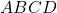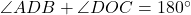How Cheenta works to ensure student success?
Explore the Back-Story

# CMI Entrance 2019 Problem from Transformation GeometryLet's discuss a problem from CMI Entrance Exam 2019 based on the Inscribed Angle Theorem or Central Angle Theorem and Transformation Geometry.

The Problem:

Letbe a parallelogram. Let 'O' be a point in its interior such that. Show that.

The Solution:

## Some useful resources:

Let's discuss a problem from CMI Entrance Exam 2019 based on the Inscribed Angle Theorem or Central Angle Theorem and Transformation Geometry.

The Problem:

Letbe a parallelogram. Let 'O' be a point in its interior such that. Show that.

The Solution:

## Some useful resources:

This site uses Akismet to reduce spam. Learn how your comment data is processed.

### Knowledge Partner